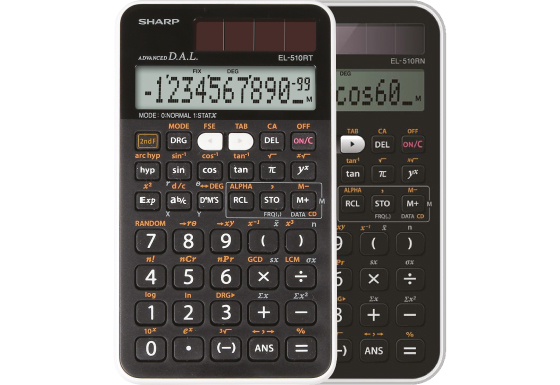## EL-510RT/RN

Calculators EL-510RN and EL-510RT have 161 and 169 built-in functions including 1-variable statistics and offers the possibility to perform calculations in DEC, BIN, OCT, HEX and PEN systems. Both models have a large 1-line display and 10+2 digits capacity, dual power and a hard cover to protect them from scratches and falls.## Specification EL-510RN

• Built-In Functions: 131
• Entry System: Algebraic (D.A.L.)
• Memory Variables: 1
• Power Source: Solar & Battery
• Dimensions and Weight: 135 × 76 × 10 mm, 66 g

Mathematical Functions

• Complex Number Calculation

Statistics

• 1-Variable Statistics
• Mean, Standard Deviation
• Random Numbers

Trigonometric Functions

• Sin, Cos, Tan and Inverses
• Hyperbolic Functions
• Sexagesimal and Decimal Conversions
• Rectangular and Polar Coordinates Conversions

Number Systems

• Number Systems: DEC, BIN, OCT, HEX, PEN

## Specification EL-510RT

• Built-In Functions: 169
• Entry System: Algebraic (D.A.L.)
• Power Source: Solar & Battery
• Dimensions and Weight: 135 × 76 × 10 mm, 66 g

Mathematical Functions

• Fractions

Memory

• Multi-Line Playback
• Memory Variables: 5

Notation and Decimal Places

• FSE Display (SCI, FIX, ENG)
• 3-Digit Punctuation

Statistics

• 1-Variable Statistics
• Mean, Standard Deviation
• Random Numbers

Trigonometric Functions